Online Math Center

# Tricks To Solve Combined Operations

Solving combined operations might cause you a headache, but in mathematics, there’s always a key that unlocks the easy way of solving problems.

In case you missed our articles on how to make math easier, check out our blog anytime for more math tips.

Before diving into combined operations, we will also discuss how to solve fractions in an easy way since they are an important part of combined math operations.

### How To Solve Fractions

In order to solve additions and subtractions with fractions, you need a common denominator. When the operated fractions already have the same denominator, we just add or subtract the numerators.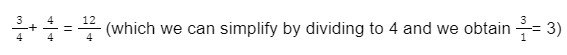When we have different denominators: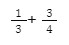The prime factorization of 3 and 4 is 12. So, we multiply the first fraction with 4 and the next one with 3 in order to obtain 12 as a common denominator, hence we will have: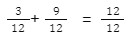Notice that we maintain the value of the denominator and add up just the numerators 3 + 9 which equals 12. Lastly, we reduce the obtained fraction to the minimum until the fraction cannot be simplified further. In this case, we divide by 12 and we will obtain: 1/1.

Did you know that the line “ – “ which divides the numerator from the denominator is called: “ Vinculum”?

### Multiplication of Fractions

Multiplying fractions might be the easiest operation. We just need to multiply the numerators by each other and the denominators by each other: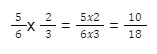If we reduce the fraction to a common divider, in this case, 2, and we will obtain: 5/9, the fraction can no longer be simplified.

We could have reduced the fraction during the first step: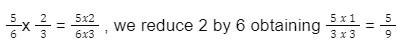### Division of Fractions

Dividing fractions is an easy operation, as well, if you remember to flip the divisor-fraction. We flip the divisor-fraction and then multiply the two fractions.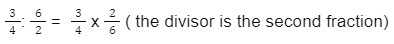Now we reduce the common numbers in the two fractions, criss-cross: 3 to 6 and 4 to 2 and we obtain:### Raising A Fraction To Power

When we raise a fraction to power, we raise both the numerator and the denominator to the same exponent.Now, if you also stumble upon a fraction in a complex operation, you will know how to reach the correct solution.

### How To Solve Combined Operations

Order is very important in solving mathematical combined operations. If you have a hard time remembering the right order of operations, keep in mind the following initials:

P

E

MD

AS

“ P “ stands for parentheses & brackets: (   ) ; [   ] ; {   }

“ E” stands for exponents, also known as powers: 34

“ MD “ stands for multiplication and division, which are always solved from left to right, whether is multiplication or division first: 3 x 4 + 1 : 1

“ AS ” stands for addition and subtraction, which are always solved from left to right, regardless of which one is the first.

This order is available for algebraic mathematics when we solve numeric operations.

There are also combined operations with sets of numbers.

In set theory, combined operations involve the intersection, union, and complement of sets. Even in set operations, we respect the following order: parentheses first, then all the other operations are performed from left to right.

#### Exercise:

Given that U = {x : 1 ≤ x ≤ 10, x is an integer}, G = {x : x is a prime number}, H = {x : x is an even number}, P = {1, 2, 3, 4, 5}. Please list the elements belonging to the following set: GHP.

You can find more exercises with combined set operations and the solution here

For now, let’s try some numeric combined operations. Repeat the order of operations before tackling these exercises:

Find the result of the following combined operations:

1. 3 x (10 + 6 – 7 + 9) = ?
2. 9 – 2 + 8 x (4 + 6) = ?
3. (7 x (4 + 3 – 6)) x 2 = ?

For more practice, you can try online math worksheets

### Math Hacks

No matter what number you choose, the answer will always be 2 to the following recipe:

1. Choose a number.

2. Multiply your number by 3.

4. Divide the number obtained at step 3 by 3.

5. Subtract the number that you chose from the number obtained at step 4

#### What is the result?

At the Online Math Center, our math tutors are ready to help you become a master of mathematics.

We offer tutoring programs for middle to high school students in groups or individually.

Contact us anytime whether you are a parent looking for math tutoring for your child or a student preparing for math competitions

## Step One

It only takes two steps to schedule a free lesson with an OMC representative.

To get started, please tell us who you are:

## Dear Parent!

Thank you for placing your trust in us to educate your child!

Congratulations on joining the Online Math Center!
See you in class.

## Thank you!

Our manager will be in contact with you shortly.

## Step Two

Let us know how to contact you. One of our representatives will get back to you shortly.

## Step Two

Awesome! We need to get in touch with your parent or guardian for further discussion. Please check in with them before filling out the form below with their information.

## Step One

It only takes two steps to schedule an appointment with an OMC representative.

To get started, please tell us who you are: